https://doi.org/10.5194/acp-21-18055-2021
https://doi.org/10.5194/acp-21-18055-2021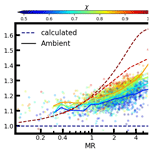# Method to quantify black carbon aerosol light absorption enhancement with a mixing state index

Gang Zhao, Tianyi Tan, Yishu Zhu, Min Hu, and Chunsheng Zhao
Abstract

Large uncertainties remain when estimating the warming effects of ambient black carbon (BC) aerosols on climate. One of the key challenges in modeling the radiative effects is predicting the BC light absorption enhancement, which is mainly determined by the mass ratio (MR) of non-BC coating material to BC in the population of BC-containing aerosols. For the same MR, recent research has found that the radiative absorption enhancements by BC are also controlled by its particle-to-particle heterogeneity. In this study, the BC mixing state index (χ) is developed to quantify the dispersion of ambient black carbon aerosol mixing states based on binary systems of BC and other non-black carbon components. We demonstrate that the BC light absorption enhancement increases with χ for the same MR, which indicates that χ can be employed as a factor to constrain the light absorption enhancement of ambient BC. Our framework can be further used in the model to study the radiative effects of black carbon on climate change.

Share
Dates
1 Introduction

Black carbon (BC) aerosols absorb solar radiation, thus exerting warming effects on the earth's energy system (Bond et al., 2006, 2013). However, large uncertainties remain when quantifying the BC warming effects (Menon et al., 2002; Koch et al., 2009; Jacobson, 2010; Cui et al., 2016). Most of the BC particles were emitted from incomplete combustion of bio-fossil fuel (Bond et al., 2013). After being initially emitted, the BC particles experience an aging process with some other non-BC components coated on the BC particles (Peng et al., 2016, 2017). During the aging process, the light absorption of BC aerosols would increase, which is well known as the “lensing effect” (Saleh et al., 2013, 2014). One critical challenge in estimating the BC warming effects is quantifying the lensing effects of ambient BC aerosols (Liu et al., 2017).

The light absorption enhancement (Eabs), which is the ratio of light absorption of BC aerosols with the coating to that of bare BC particles, is proposed to quantify the lensing effects. Comprehensive studies have been carried out to study the Eabs (Cappa et al., 2012; Liu et al., 2015; Fierce et al., 2016; Peng et al., 2016; Liu et al., 2017; Fierce et al., 2020). However, a large discrepancy remains between the results of Eabs from field measurements and laboratory studies. The measured Eabs of laboratory-generated monodisperse BC particles can reach up to a factor of 2, which is consistent with the results from the Mie scattering model (Cappa et al., 2012, 2019). However, some field measurement shows that the Eabs values of ambient BC aerosols are relatively small, with 1.06 at California (Cappa et al., 2012), 1.07 in South China (Lan et al., 2013), and 1.10 in Japan (Nakayama et al., 2014), while the measured Eabs of ambient BC reaches 1.59 during summer time in Beijing (Xie et al., 2019).

Many factors, such as the morphology of the BC core, the position of BC core inside coating, the coating thickness, chemical properties of coating materials, and size distribution of the BC, influence the Eabs of ambient BC aerosols. Wu et al. (2018) reported that the BC light absorption properties vary significantly for different morphology from the calculation of models. Laboratory studies also find that the light absorption properties of the BC core were tuned due to the change of the BC core morphology (Yuan et al., 2020). Compared with the concentric spherical structure, the off-center coated BC aggregates would lead to up to a 31 % reduction in Eabs by the multiple-sphere T-matrix method (Zhang et al., 2017). It has been well studied that the Eabs is highly related with the mass ratio (MR) of coating materials and BC core (Liu et al., 2014, 2017). The coating materials are also critical in regulating the morphology and optical properties as the coating of sulfuric acid has been shown to be more efficient in altering the BC morphology and light absorption (Zhang et al., 2008; Xue et al., 2009b, a). Zhao et al. (2019b) reported that the light absorption properties of ambient BC particles are influenced by BC mass size distribution. In addition, recently, researchers have found that the Eabs values are also controlled by particle-to-particle heterogeneity (Fierce et al., 2016, 2020). As shown in Fig. 1, the Eabs of ambient aerosols for the same MR varies by about 30 %, which is consistent with the results of Fierce et al. (2020). However, there is no study, to the best of our knowledge, that constrains the uncertainties of the Eabs for the same MR.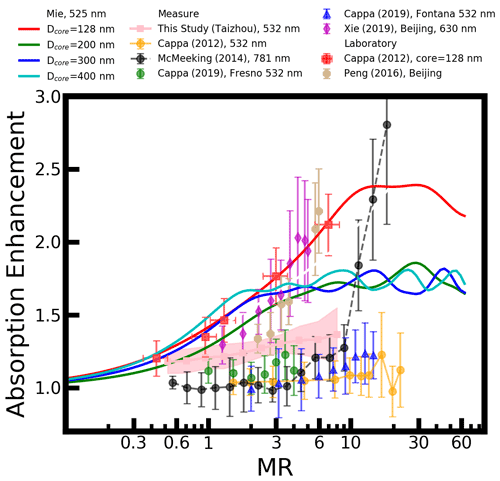Figure 1The measured Eabs of BC particles from different ambient measurements, including this work (in pink) and lab studies.

In this study, we developed a BC mixing state index (χ) to quantify the dispersion of black carbon aerosol mixing states based on binary systems of BC and other non-black carbon components. We demonstrate that the BC Eabs increases with χ for the same MR based on the field measurement, which indicates that χ can be employed as a factor to constrain the Eabs properties of ambient BC.

2 Data and methods

## 2.1 Field measurements

The field measurements were conducted at a suburban site in Taizhou (11957 E, 3235 N) from 26 May to 18 June. As shown in Fig. S1, the Taizhou site lies between two large cities of Nanjing and Shanghai, where the aerosols can be seen as representative of those of the Yangtze River Delta area (Liu et al., 2020). For more details of the field measurements, the reader is referred to Zhao et al. (2019a). During the field measurements, we placed all of the instruments in a container where the temperature was carefully controlled between 22 and 26 C. A PM10 impactor, which is about 5 m above the ground, was mounted on the top of the container. The sample aerosols were drawn from the impactor and then dried by a Nafion dryer tube.

The size-resolved BC core distribution and non-BC coating thickness were measured using a differential mobility analyzer (DMA, model 3081, TSI, USA) in tandem with a single-particle soot photometer (SP2, Droplet Measurement Technologies, USA). For detailed information on the DMA, the reader is referred to Zhao et al. (2019c). SP2 can measure the BC mass concentration from the incandescence signals emitted by the BC particle, which is heated to around 6000 K by a laser with a wavelength of 1064 nm (Zhao et al., 2020b). Along with the measurement of size-resolved BC distributions, a nephelometer (Aurora 300, Ecotech, Australia) (Müller et al., 2011) was employed to measure the aerosol scattering coefficient (σsca) at the wavelength of 525 nm.

## 2.2 BC mixing states from the DMA–SP2 system

In this study, the SP2 was placed behind the DMA to measure the size-selected distribution of BC core and non-BC coating thickness. The schematic instrument setup is shown in Fig. S2, and the reader is referred to Sect. 2 in the Supplement for details. The DMA was set to scan the aerosols' Dp from 12.3 to 697 nm over a period of 285 s and repeated after a pause of 15 s. After careful calibrations of the SP2 (Sect. 3.1 in the Supplement), transformations of the measured signals to BC mass concentrations (Sect. 3.2 in the Supplement), and multiple charging corrections (Sect. 3.3 in the Supplement), the BC-containing number concentration distribution under different total diameter (Dp) and BC core diameter (Dc) values can be calculated, as shown in Fig. S5b. For the details of the calculation of the size-resolved distribution of BC core and coating thickness from the DMA-SP2 system, the reader is referred to Zhao et al. (2020a). The measured size-resolved distribution of BC core and coating thickness as in Fig. S5b were used for further analysis. It should be mentioned that the measured number distribution of BC-containing aerosols is two-dimensional $\left(\frac{{d}^{\mathrm{2}}N}{\mathrm{dlogDp}\cdot \mathrm{dlogDc}}\right)$. As noted by Zhao et al. (2020b), the SP2 can only detect these BC-containing aerosols with a core diameter larger than 84 nm. The DMA selects the aerosol in the range between 13.3 and 749.9 nm. In the following discussion, the size-resolved distribution of BC core and coating thickness is constrained in the range between 84 and 697 nm.

## 2.3 Calculating the aerosol optical properties

### 2.3.1 Calculating the single-particle aerosol absorption coefficient for a given Dp and Dc

A Mie scattering core–shell model (Bohren et al., 2007) was employed to calculate the aerosol absorption coefficient (σabs). When calculating the σabs of single particles, the Mie scattering model requires the diameter of the core, the coating thickness, the refractive index of the core, and the refractive index of the shell. The refractive index of the core adopted here is 1.67+0.67i, which is the mean value calculated by comparing the measured light absorption and calculated light absorption properties (Zhao et al., 2020a). The refractive index of the shell is chosen to be 1.46+0i, which is assumed to be that of the non-BC component measured by the DMA-SP2 system (Zhao et al., 2019a, c). With the above information, the σabs values at a given Dp and a given Dc can be calculated.

### 2.3.2 Calculating the aerosol bulk absorption coefficient

We calculate the single-particle σabs of different Dp and Dc with the given refractive index of core and shell, and then the ambient aerosol σabs distributions at different Dp and Dc $\left(\frac{{d}^{\mathrm{2}}{\mathit{\sigma }}_{\mathrm{abs}}}{\mathrm{dlogDp}\cdot \mathrm{dlogDc}}\right)$ can be calculated by multiplying the number concentrations of the BC-contained aerosols $\left(\frac{{d}^{\mathrm{2}}N}{\mathrm{dlogDp}\cdot \mathrm{dlogDc}}\right)$. By integrating the $\frac{{d}^{\mathrm{2}}{\mathit{\sigma }}_{\mathrm{abs}}}{\mathrm{dlogDp}\cdot \mathrm{dlogDc}}$ over different Dc values, the ambient aerosol σabs distribution along with different Dp $\left(\frac{\mathrm{d}{\mathit{\sigma }}_{\mathrm{abs}}}{\mathrm{dlogDp}}\right)$ can be calculated. The total σabs of the ambient BC-containing aerosols can be calculated by integrating the $\frac{\mathrm{d}{\mathit{\sigma }}_{\mathrm{abs}}}{\mathrm{dlogDp}}$ over different Dp values.

### 2.3.3 Calculating the aerosol Eabs

Along with calculating the σabs(DpDc) of single particles for different Dp and Dc, we calculate the corresponding light absorption (σabs(DcDc)) value for Dc without thickness. The corresponding total light absorption of all measured BC-contained aerosols without coating can be calculated by integrating the calculated σabs(DcDc) among different Dp and Dc weighted with $\frac{{d}^{\mathrm{2}}N}{\mathrm{dlogDp}\cdot \mathrm{dlogDc}}$. Thus the ambient BC particles without coating (σabs(Dp=Dc)) can be calculated. The bulk ambient aerosol Eabs can thus be calculated with ${E}_{\mathrm{abs}}=\frac{{\mathit{\sigma }}_{\mathrm{abs}}}{{\mathit{\sigma }}_{\mathrm{abs}}\left({D}_{\mathrm{p}}={D}_{\mathrm{c}}\right)}$.

## 2.4 Quantifying BC mixing states

In this study, the mass-weighted mixing state index for BC-containing particles (χ) is developed to investigate the distribution of non-BC material across the BC-containing particle population, which is essentially the same as that of Yu et al. (2020). As for BC particles with known Dp and Dc, the mass concentration of BC core and coating material can be calculated with the effective density of BC core and coating material. The effective density of the BC core is calculated in detail in Sect. 2.2 in the Supplement. The effective density of the coating material is assumed to be the same as the measured effective density of non-BC aerosols using a centrifugal particle mass analyzer (version 1.53, Cambustion Ltd, UK) in tandem with a scanning mobility particle sizer system (Zhao et al., 2019a), and a mean value of 1.5 g/cm3 was used here.

For each particle i(i= 1, 2, …, N is the measured BC-containing aerosol number concentration), we can calculate its mass ratio of BC with

$\begin{array}{}\text{(1)}& {p}_{i,\mathrm{BC}}=\frac{{m}_{i,\mathrm{BC}}}{{m}_{i}},\end{array}$

where mi,BC is the mass concentration of BC, and mi is the total mass concentration of particle i. The mass portion of BC can be calculated as

$\begin{array}{}\text{(2)}& {p}_{\mathrm{BC}}=\frac{{m}_{\mathrm{BC}}}{{m}_{\mathrm{tot}}},\end{array}$

where mBC (the total mass concentration of BC) and mtot (total mass of BC-containing aerosols) can be calculated as ${m}_{\mathrm{BC}}={\sum }_{i=\mathrm{1}}^{N}{m}_{i,\mathrm{BC}}$, ${m}_{\mathrm{tot}}={\sum }_{i=\mathrm{1}}^{N}{m}_{i}$. The MR is calculated as

$\begin{array}{}\text{(3)}& \mathrm{MR}=\frac{\left({m}_{\mathrm{tot}}-{m}_{\mathrm{BC}}\right)}{{m}_{\mathrm{BC}}}.\end{array}$

The mass portion of particle i to total BC-containing aerosols is calculated as

$\begin{array}{}\text{(4)}& {p}_{i}=\frac{{m}_{i}}{{m}_{\mathrm{tot}}}.\end{array}$

With the definition above, we can calculate the mixing entropy of particle i(Hi) by

$\begin{array}{}\text{(5)}& {H}_{i}=-\left({p}_{i,\mathrm{BC}}ln\left({p}_{i,\mathrm{BC}}\right)+\left(\mathrm{1}-{p}_{i,\mathrm{BC}}\right)\mathrm{ln}\left(\mathrm{1}-{p}_{i,\mathrm{BC}}\right),\end{array}$

the average mixing entropy of the population by

$\begin{array}{}\text{(6)}& {H}_{\mathit{\alpha }}={\sum }_{i=\mathrm{1}}^{N}{p}_{i}{H}_{i},\end{array}$

and the population bulk mixing entropy by

$\begin{array}{}\text{(7)}& {H}_{\mathit{\gamma }}=-\left({p}_{\mathrm{BC}}\mathrm{ln}\left({p}_{\mathrm{BC}}\right)+\left(\mathrm{1}-{p}_{\mathrm{BC}}\right)ln\left(\mathrm{1}-{p}_{\mathrm{BC}}\right).\end{array}$

Then the average particle species diversity can be calculated by

$\begin{array}{}\text{(8)}& {D}_{\mathit{\alpha }}={e}^{{H}_{\mathit{\alpha }}},\end{array}$

and the bulk population species diversity can be calculated by

$\begin{array}{}\text{(9)}& {D}_{\mathit{\gamma }}={e}^{{H}_{\mathit{\gamma }}}.\end{array}$

With the above information, the dispersion of BC particle mixing states can be defined as

$\begin{array}{}\text{(10)}& \mathit{\chi }=\frac{{D}_{\mathit{\alpha }}-\mathrm{1}}{{D}_{\mathit{\gamma }}-\mathrm{1}}.\end{array}$

The basic idea of quantifying the BC particle mixing states is the same as that of Riemer et al. (2013) and Riemer et al. (2019); their framework mainly focuses on the bulk ambient aerosols with about five species (Bondy et al., 2018; Ye et al., 2018). Several different (binary) species definitions for χ have been used in the literature. Ching et al. (2017) used this index to study the impact of mixing of hygroscopic and non-hygroscopic species on cloud condensation nuclei. Dickau et al. (2016) quantified the volatile and nonvolatile species mixing characters. Zheng et al. (2021) compared three different variants for χ, one of which was based on absorbing (BC) and non-absorbing species, and Yu et al. (2020) used a metric that is very related to this paper. Our developed χ is a reduced parameter that only concerns the BC-containing aerosols with two species of BC component and non-BC coating materials.

3 Results and discussions

## 3.1 BC mixing state diagram

A mixing state diagram as shown in Fig. 2 was employed for better understanding of the dispersion of BC mixing states. Nine different aerosol populations are given and summarized in Table 1. For each group, we include six BC-containing particles with different mass concentrations of BC core and non-BC coating material.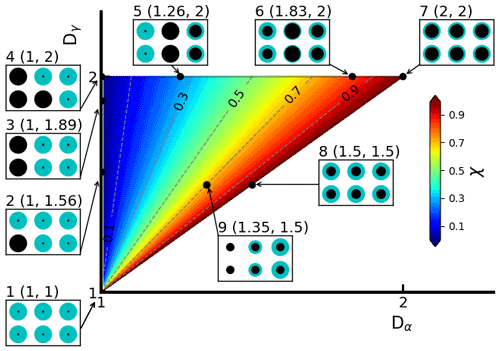Figure 2Mixing state diagram to illustrate the relationship between Dα, Dγ, and χ. Each species consists of six particles, and the colors of black and cyan represent the BC and non-BC components.

Table 1Detailed information of the BC particles shown in Fig. 2.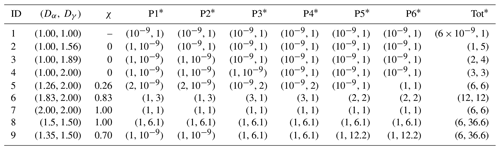Mass of the BC component and non-BC component (arbitrary units).

For group 1, the amounts of BC are very small (near zero), and most of the aerosols are composed of the non-BC component. The Dα and Dγ values are 1.00 and 1.00 respectively. These groups can also be described as all of the particles are pure BC particles without coating.

For groups 2, 3, and 4, the mass concentration ratios of the BC component to the non-BC component are 1 : 5, 2 : 4, and 3 : 3 respectively. All of the Dα values are 1.00 for groups 2, 3, and 4 because the BC particles are externally mixed. The corresponding Dγ values are 1.56, 1.89, and 2.00 respectively. For these three groups, the χ values are all 0.00.

For groups 4, 5, 6, and 7, the mass concentration ratios of the BC component to the non-BC component are all 1 : 1, while the BC component is mixed to a different extent. It is easy to conclude that the BC particles of group 7 are most well mixed among these four groups. The corresponding χ values are 0, 0.26, 0.83, and 1.0 for group 4, 5, 6, and 7, respectively.

As for groups 8 and 9, the mass concentration ratios of the BC component to the non-BC component are 1 : 6.1. The Dγ values are 1.5, and the Dα values are 1.5 and 1.35 respectively.

From the different groups, the average particle species diversity Dγ value is mainly determined by the total mass concentration ratio of the BC component to the non-BC component. It varies between 1 and 2 for different total mass concentration ratios. The Dγ increases when the mass ratio approaches 1. The bulk population species diversity Dα ranges between 1 and Dγ. It denotes the diversity of different BC-containing particles.

## 3.2 Overview of the measurements

Figure S6 gives the time series of our field measurement results. During the field measurements, the σsca varies between 29 and 1590 Mm−1. The ranges of Hα, Hγ, Dα, Dγ, and χ are 0.10–0.55, 0.42–0.64, 1.32–1.72, 1.52–1.91, and 0.62–0.82 respectively.

For a better understanding of the characteristics of the above parameters, we only present the time series of these parameters during a pollution period between 27 and 30 May in Fig. 3. As shown in Fig. 3, the MR increased from about 2 to 4 when the σsca increased from 300 to 1200 Mm−1, which indicates that some secondary aerosol components were coated on the BC particles when the ambient air is more polluted. During the aging process, the Hα decreased from 0.51 to 0.38 and Hγ decreased from 0.63 to 0.49. The Dα decreases from 1.66 to 1.48. The Dγ decreases with the MR from 1.86 to 1.66, which is consistent with the results in Sect. 3.1 that the Dγ should decrease with the MR when the MR is larger than 1. The χ varies between 0.68 and 0.79. It is worth noting that the χ is not well correlated with the pollution conditions.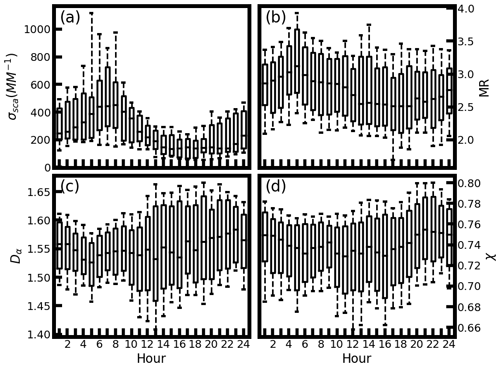Figure 4Daily variation of the measured (a) σsca, (b) MR, (c) Dα, and (d) χ.

The corresponding mean values of BC-containing number size distributions under different Dp and Dc between the days of 27 and 28, 28 and 29, and 29 and 30 May are shown in Fig. S7. It is obvious that the BC-containing number and coating thickness increase with the pollution levels. However, the normalized BC core distributions are almost the same for different pollution levels as shown in Fig. S8. The daily variation of σsca, which is highly related to the development of the boundary layer, reaches its maximum value of 525 Mm−1 at 06:00 and a minimum value of 150 Mm at 19:00, as shown in Fig 4. The daily variation of MR is largest at 05:00, with a mean value of 3.16, and reaches its minimum value of 2.56 at 19:00. The daily variation of MR was mainly influenced by the aging process and anthropogenic activities. During the daytime, the newly emitted BC particles due to anthropogenic activities have low MR, and the measured mean MR is lower than that at night. The Dα values, which are anti-correlated with MR, show the opposite trend with MR. As for χ, it is smaller in the daytime than that at night. The lower χ values in the daytime mainly resulted from the mixing of newly emitted BC particles due to anthropogenic activities and some preexisting aged BC particles.

## 3.3 Relationship between the χ and Eabs from measurements

For each of the measured group of size-resolved distribution of BC core and coating thickness, we calculated the corresponding MR, χ, and Eabs. And the relationship between the MR and absorption enhancement is summarized in Fig. 5. It should be noted that the shown BC population is only one of the possible examples with χ equaling 0, 0.81, and 1 respectively. There are many other possible ways the particle composition can be arranged that would give the same mixing state index.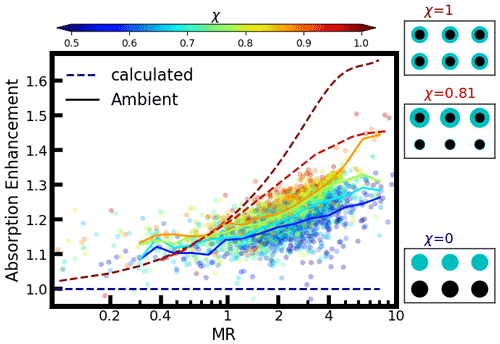Figure 5Relationship between the BC Eabs and the measured mass ratio of the BC-containing aerosols coating material to BC under different χ conditions. The four solid lines from bottom to top correspond to the measured ambient size-resolved BC mixing states data with χ ranges of 0.575–0.625, 0.625–0.675, 0.675–0.725, and 0.725–0.775. The dotted line corresponds to χ values of 0.0 (blue), 0.81 (light red), and 1.0 (dark red), respectively.

Overall, the BC Eabs values increase with MR, which is consistent with previous knowledge. For a given value of MR, Eabs varies by about 20 %, especially for these conditions with MR larger than 1.0. When MR is larger than 1.0, the Eabs increases with the χ. The relationship between the Eabs and χ is rather complex when MR is smaller than 1.0. However, only 448 of 6948 groups (6.4 %) of the measured MR values are smaller than 1. Therefore, for most of the conditions, the measured Eabs should increase with χ, which indicates that the BC mixing state index χ can be employed as a factor to constrain the Eabs of ambient aerosols.

A schematic diagram as shown in Fig. 6 to denote the relationship between the Eabs and χ. From Fig. 6, we calculated the Eabs and χ under different MRs and then compared the Eabs of different bulk aerosols. The first group contains two particles with both the MRs equaling 8. The corresponding χ is 1.00, and Eabs is 1.60. Another group of particles contains two particles with MRs equaling 1 and 15, respectively. Thus the second group of particles has a mean MR of 8. The calculated corresponding χ and Eabs are 0.79 and 1.42 respectively. Thus, the Eabs tends to increase with χ for the same MR, which mainly results from the increasing ratio of Eabs (the slope of Eabs to MR) decreasing with MR.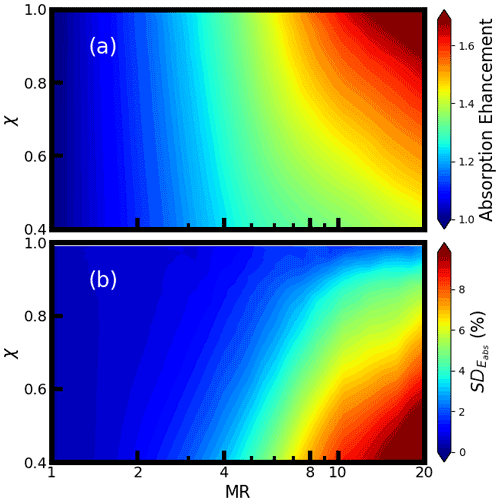Figure 7The calculated (a) mean Eabs values and (b) standard deviations of the Eabs values for different MR and χ.

It is worth noting that the increasing ratio is almost the same when the MR is in the range of 0 and 3. Therefore, the Eabs does not tend to increase with the χ when the MR is less than 1, which is consistent with our study, as shown in Fig. 6.

## 3.4 Relationship between the χ and Eabs from simulations

A Monte Carlo simulation was carried out for a better understanding of the relationship between χ and Eabs. During the simulation, a group of the BC-containing aerosols was generated with the Dp and Dc meeting the following conditions, and the number of BC-containing particles was assumed to be 30. For each of the BC-containing particles, the core diameter of the BC particle was randomly generated with a geometric mean diameter of 130.7 nm and a geometric standard deviation of 1.5, which are the mean measurement results of the BC core distribution during the field measurements (Zhao et al., 2020b). The corresponding MR of the BC particle is assumed to be randomly distributed in the range between 0.0 (pure BC particles without coating) and 78.0 (particles with a core diameter of 130 nm and a total diameter of 560 nm). For each group of particles, the corresponding aerosol bulk MR, Eabs, and χ can be calculated using the core–shell Mie scattering model and the parameterization proposed by Wu et al. (2018) to account for the non-sphericity of the BC aerosols. The simulations were conducted 107 times, and the calculated mean and standard deviation of Eabs under different MR and χ are summarized in Fig. 7a and b.

From Fig. 7a, the calculated Eabs tends to increase with MR for each of the given χ values, which is consistent with previous knowledge of the BC light absorption properties. When the MR is smaller than 2, the calculated Eabs does not seem to increase with the χ, which is consistent with the analyzed results from Sect. 3.3 and Fig. 6. When the MR is larger 2, the Eabs tends to increase with the χ. The larger the MR is, the more sensitive Eabs is to χ. There may be two reasons for this phenomenon. One reason is that the calculated slope of Eabs to MR for one particle as shown in Fig. 6 decreases with the MR. Another reason is that the calculated Eabs range increases with MR when the χ changes between 0 and 1 as shown in Fig. 5.

As for the uncertainties of simulated Eabs, it tends to increase with the MR, which is consistent with the previous discussions that the Eabs range tends to increase with MR. Overall, the calculated standard deviations of Eabs are smaller than 10 % for different MR and χ. Therefore, the calculated Eabs can be well constrained by χ. When the ambient aerosol χ and MR are measured, the corresponding Eabs can be estimated from Fig. 7a.

4 Conclusion

Larger uncertainties remain when estimating the warming effects of ambient BC aerosols due to the poor understanding of the ambient BC light absorption enhance ratio. Previous studies find that the light absorption of ambient aerosols was mainly determined by the morphology of the BC core, the position of the BC core inside coating, the coating thickness, and the size distribution of the BC. We find that there are more than 20 % of uncertainties for the same measured mean coating thickness, i.e. the same measured MR based on the field measurements of the size-resolved distribution of BC core and coating thickness. However, there was no study until now, to the best of our knowledge, that attempts to constrain the uncertainties.

In this study, we developed the BC mixing state index χ based on the mass concentrations of BC components and non-BC material of each BC-containing particle. Results show that the light absorption enhancement ratio Eabs tend to increase the χ for the same measured MR. Therefore, our developed parameter χ, which reflects the dispersion of the BC mixing states, can be employed as an effective parameter to constrain the light absorption enhancement of ambient BC-containing aerosols.

The new finding of our study is that the mixing state index can contribute to improvements in the accuracy of simulating the BC radiative effects. In the particle-resolved simulation of ambient aerosols, the particle-to-particle heterogeneity of BC-containing aerosols can be resolved by simply introducing the BC mixing state index χ. The aerosol light absorption enhancement can be better constrained by MR and χ, and then the radiative effects of BC can be estimated. Therefore, our framework can be employed in the model by simply introducing a BC mixing state index for better estimating the BC radiative effects.

Data availability

The research data are available within the paper.

Supplement

Author contributions

GZ wrote the manuscript. CZ, MH, TT, SG, ZW, YZ, and GZ discussed the results.

Competing interests

The contact author has declared that neither they nor their co-authors have any competing interests.

Disclaimer

Publisher’s note: Copernicus Publications remains neutral with regard to jurisdictional claims in published maps and institutional affiliations.

Financial support

This research has been supported by the China Postdoctoral Science Foundation (grant no. 2021M700192), the National Natural Science Foundation of China (grant no. 41590872), and the National Key R&D Program of China (grant no. 2016YFC020000: Task 5).

Review statement

This paper was edited by Manvendra K. Dubey and reviewed by three anonymous referees.

References

Bohren, C. F. and Huffman, D. R.: Absorption and Scattering by a Sphere, in: Absorption and Scattering of Light by Small Particles, Wiley-VCH Verlag GmbH, 82–129, https://doi.org/10.1002/9783527618156, 2007.

Bond, T. C. and Bergstrom, R. W.: Light Absorption by Carbonaceous Particles: An Investigative Review, Aerosol Sci. Tech., 40, 27–67, https://doi.org/10.1080/02786820500421521, 2006.

Bond, T. C., Doherty, S. J., Fahey, D. W., Forster, P. M., Berntsen, T., DeAngelo, B. J., Flanner, M. G., Ghan, S., Karcher, B., Koch, D., Kinne, S., Kondo, Y., Quinn, P. K., Sarofim, M. C., Schultz, M. G., Schulz, M., Venkataraman, C., Zhang, H., Zhang, S., Bellouin, N., Guttikunda, S. K., Hopke, P. K., Jacobson, M. Z., Kaiser, J. W., Klimont, Z., Lohmann, U., Schwarz, J. P., Shindell, D., Storelvmo, T., Warren, S. G., and Zender, C. S.: Bounding the role of black carbon in the climate system: A scientific assessment, J. Geophys. Res.-Atmos., 118, 5380–5552, https://doi.org/10.1002/jgrd.50171, 2013.

Bondy, A. L., Bonanno, D., Moffet, R. C., Wang, B., Laskin, A., and Ault, A. P.: The diverse chemical mixing state of aerosol particles in the southeastern United States, Atmos. Chem. Phys., 18, 12595–12612, https://doi.org/10.5194/acp-18-12595-2018, 2018.

Cappa, C. D., Onasch, T. B., Massoli, P., Worsnop, D. R., Bates, T. S., Cross, E. S., Davidovits, P., Hakala, J., Hayden, K. L., Jobson, B. T., Kolesar, K. R., Lack, D. A., Lerner, B. M., Li, S. M., Mellon, D., Nuaaman, I., Olfert, J. S., Petaja, T., Quinn, P. K., Song, C., Subramanian, R., Williams, E. J., and Zaveri, R. A.: Radiative Absorption Enhancements Due to the Mixing State of Atmospheric Black Carbon, Science, 337, 1078–1081, https://doi.org/10.1126/science.1223447, 2012.

Cappa, C. D., Zhang, X., Russell, L. M., Collier, S., Lee, A. K. Y., Chen, C.-L., Betha, R., Chen, S., Liu, J., Price, D. J., Sanchez, K. J., McMeeking, G. R., Williams, L. R., Onasch, T. B., Worsnop, D. R., Abbatt, J., and Zhang, Q.: Light Absorption by Ambient Black and Brown Carbon and its Dependence on Black Carbon Coating State for Two California, USA, Cities in Winter and Summer, J. Geophys. Res.-Atmos., 124, 1550–1577, https://doi.org/10.1029/2018jd029501, 2019.

Ching, J., Fast, J., West, M., and Riemer, N.: Metrics to quantify the importance of mixing state for CCN activity, Atmos. Chem. Phys., 17, 7445–7458, https://doi.org/10.5194/acp-17-7445-2017, 2017.

Cui, X., Wang, X., Yang, L., Chen, B., Chen, J., Andersson, A., and Gustafsson, Ö.: Radiative absorption enhancement from coatings on black carbon aerosols, Sci. Total Environ., 551–552, 51–56, doi.org/10.1016/j.scitotenv.2016.02.026, 2016.

Dickau, M., Olfert, J., Stettler, M. E. J., Boies, A., Momenimovahed, A., Thomson, K., Smallwood, G., and Johnson, M.: Methodology for quantifying the volatile mixing state of an aerosol, Aerosol Sci. Tech., 50, 759–772, https://doi.org/10.1080/02786826.2016.1185509, 2016.

Fierce, L., Bond, T. C., Bauer, S. E., Mena, F., and Riemer, N.: Black carbon absorption at the global scale is affected by particle-scale diversity in composition, Nat. Commun., 7, 12361, https://doi.org/10.1038/ncomms12361, 2016.

Fierce, L., Onasch, T. B., Cappa, C. D., Mazzoleni, C., China, S., Bhandari, J., Davidovits, P., Fischer, D. A., Helgestad, T., Lambe, A. T., Sedlacek III, A. J., Smith, G. D., and Wolff, L.: Radiative absorption enhancements by black carbon controlled by particle-to-particle heterogeneity in composition, P. Natl. Acad. Sci. USA, 117, 5196–5203, https://doi.org/10.1073/pnas.1919723117, 2020.

Jacobson, M. Z.: Short-term effects of controlling fossil-fuel soot, biofuel soot and gases, and methane on climate, Arctic ice, and air pollution health, J. Geophys. Res.-Atmos., 115, D14209, https://doi.org/10.1029/2009JD013795, 2010.

Koch, D., Schulz, M., Kinne, S., McNaughton, C., Spackman, J. R., Balkanski, Y., Bauer, S., Berntsen, T., Bond, T. C., Boucher, O., Chin, M., Clarke, A., De Luca, N., Dentener, F., Diehl, T., Dubovik, O., Easter, R., Fahey, D. W., Feichter, J., Fillmore, D., Freitag, S., Ghan, S., Ginoux, P., Gong, S., Horowitz, L., Iversen, T., Kirkevåg, A., Klimont, Z., Kondo, Y., Krol, M., Liu, X., Miller, R., Montanaro, V., Moteki, N., Myhre, G., Penner, J. E., Perlwitz, J., Pitari, G., Reddy, S., Sahu, L., Sakamoto, H., Schuster, G., Schwarz, J. P., Seland, Ø., Stier, P., Takegawa, N., Takemura, T., Textor, C., van Aardenne, J. A., and Zhao, Y.: Evaluation of black carbon estimations in global aerosol models, Atmos. Chem. Phys., 9, 9001–9026, https://doi.org/10.5194/acp-9-9001-2009, 2009.

Lan, Z.-J., Huang, X.-F., Yu, K.-Y., Sun, T.-L., Zeng, L.-W., and Hu, M.: Light absorption of black carbon aerosol and its enhancement by mixing state in an urban atmosphere in South China, Atmos. Environ., 69, 118–123, https://doi.org/10.1016/j.atmosenv.2012.12.009, 2013.

Liu, D., Allan, J. D., Young, D. E., Coe, H., Beddows, D., Fleming, Z. L., Flynn, M. J., Gallagher, M. W., Harrison, R. M., Lee, J., Prevot, A. S. H., Taylor, J. W., Yin, J., Williams, P. I., and Zotter, P.: Size distribution, mixing state and source apportionment of black carbon aerosol in London during wintertime, Atmos. Chem. Phys., 14, 10061–10084, https://doi.org/10.5194/acp-14-10061-2014, 2014.

Liu, D., Whitehead, J., Alfarra, M. R., Reyes-Villegas, E., Spracklen, Dominick V., Reddington, C. L., Kong, S., Williams, P. I., Ting, Y.-C., Haslett, S., Taylor, J. W., Flynn, M. J., Morgan, W. T., McFiggans, G., Coe, H., and Allan, J. D.: Black-carbon absorption enhancement in the atmosphere determined by particle mixing state, Nat. Geosci., 10, 184–188, https://doi.org/10.1038/ngeo2901, 2017.

Liu, J., Li, X., Li, D., Xu, R., Gao, Y., Chen, S., Liu, Y., Zhao, G., Wang, H., Wang, H., Lou, S., Chen, M., Hu, J., Lu, K., Wu, Z., Hu, M., Zeng, L., and Zhang, Y.: Observations of glyoxal and methylglyoxal in a suburban area of the Yangtze River Delta, China, Atmos. Environ., 238, 117727, https://doi.org/10.1016/j.atmosenv.2020.117727, 2020.

Liu, S., Aiken, A. C., Gorkowski, K., Dubey, M. K., Cappa, C. D., Williams, L. R., Herndon, S. C., Massoli, P., Fortner, E. C., Chhabra, P. S., Brooks, W. A., Onasch, T. B., Jayne, J. T., Worsnop, D. R., China, S., Sharma, N., Mazzoleni, C., Xu, L., Ng, N. L., Liu, D., Allan, J. D., Lee, J. D., Fleming, Z. L., Mohr, C., Zotter, P., Szidat, S., and Prevot, A. S.: Enhanced light absorption by mixed source black and brown carbon particles in UK winter, Nat. Commun., 6, 8435, https://doi.org/10.1038/ncomms9435, 2015.

Menon, S., Hansen, J., Nazarenko, L., and Luo, Y.: Climate effects of black carbon aerosols in China and India, Science, 297, 2250–2253, https://doi.org/10.1126/science.1075159, 2002.

Müller, T., Laborde, M., Kassell, G., and Wiedensohler, A.: Design and performance of a three-wavelength LED-based total scatter and backscatter integrating nephelometer, Atmos. Meas. Tech., 4, 1291–1303, https://doi.org/10.5194/amt-4-1291-2011, 2011.

Nakayama, T., Ikeda, Y., Sawada, Y., Setoguchi, Y., Ogawa, S., Kawana, K., Mochida, M., Ikemori, F., Matsumoto, K., and Matsumi, Y.: Properties of light-absorbing aerosols in the Nagoya urban area, Japan, in August 2011 and January 2012: Contributions of brown carbon and lensing effect, J. Geophys. Res.-Atmos., 119, 12721–712739, https://doi.org/10.1002/2014JD021744, 2014.

Peng, J., Hu, M., Guo, S., Du, Z., Zheng, J., Shang, D., Levy Zamora, M., Zeng, L., Shao, M., Wu, Y.-S., Zheng, J., Wang, Y., Glen, C. R., Collins, D. R., Molina, M. J., and Zhang, R.: Markedly enhanced absorption and direct radiative forcing of black carbon under polluted urban environments, P. Natl. Acad. Sci. USA, 113, 4266–4271, https://doi.org/10.1073/pnas.1602310113, 2016.

Peng, J., Hu, M., Guo, S., Du, Z., Shang, D., Zheng, J., Zheng, J., Zeng, L., Shao, M., Wu, Y., Collins, D., and Zhang, R.: Ageing and hygroscopicity variation of black carbon particles in Beijing measured by a quasi-atmospheric aerosol evolution study (QUALITY) chamber, Atmos. Chem. Phys., 17, 10333–10348, https://doi.org/10.5194/acp-17-10333-2017, 2017.

Riemer, N. and West, M.: Quantifying aerosol mixing state with entropy and diversity measures, Atmos. Chem. Phys., 13, 11423–11439, https://doi.org/10.5194/acp-13-11423-2013, 2013.

Riemer, N., Ault, A. P., West, M., Craig, R. L., and Curtis, J. H.: Aerosol Mixing State: Measurements, Modeling, and Impacts, Rev. Geophys., 57, 187–249, https://doi.org/10.1029/2018rg000615, 2019.

Saleh, R., Hennigan, C. J., McMeeking, G. R., Chuang, W. K., Robinson, E. S., Coe, H., Donahue, N. M., and Robinson, A. L.: Absorptivity of brown carbon in fresh and photo-chemically aged biomass-burning emissions, Atmos. Chem. Phys., 13, 7683–7693, https://doi.org/10.5194/acp-13-7683-2013, 2013.

Saleh, R., Robinson, E. S., Tkacik, D. S., Ahern, A. T., Liu, S., Aiken, A. C., Sullivan, R. C., Presto, A. A., Dubey, M. K., Yokelson, R. J., Donahue, N. M., and Robinson, A. L.: Brownness of organics in aerosols from biomass burning linked to their black carbon content, Nat. Geosci., 7, 647, https://doi.org/10.1038/ngeo2220, 2014.

Wu, Y., Cheng, T., Liu, D., Allan, J. D., Zheng, L., and Chen, H.: Light Absorption Enhancement of Black Carbon Aerosol Constrained by Particle Morphology, Environ. Sci. Technol., 52, 6912–6919, https://doi.org/10.1021/acs.est.8b00636, 2018.

Xie, C., Xu, W., Wang, J., Liu, D., Ge, X., Zhang, Q., Wang, Q., Du, W., Zhao, J., Zhou, W., Li, J., Fu, P., Wang, Z., Worsnop, D., and Sun, Y.: Light absorption enhancement of black carbon in urban Beijing in summer, Atmos. Environ., 213, 499–504, https://doi.org/10.1016/j.atmosenv.2019.06.041, 2019.

Xue, H., Khalizov, A. F., Wang, L., Zheng, J., and Zhang, R.: Effects of coating of dicarboxylic acids on the mass-mobility relationship of soot particles, Environ. Sci. Technol., 43, 2787–2792, 2009a.

Xue, H., Khalizov, A. F., Wang, L., Zheng, J., and Zhang, R.: Effects of dicarboxylic acid coating on the optical properties of soot, Phys. Chem. Chem. Phys., 11, 7869–7875, https://doi.org/10.1039/B904129J, 2009b.

Ye, Q., Gu, P., Li, H. Z., Robinson, E. S., Lipsky, E., Kaltsonoudis, C., Lee, A. K. Y., Apte, J. S., Robinson, A. L., Sullivan, R. C., Presto, A. A., and Donahue, N. M.: Spatial Variability of Sources and Mixing State of Atmospheric Particles in a Metropolitan Area, Environ. Sci. Technol., 52, 6807–6815, https://doi.org/10.1021/acs.est.8b01011, 2018.

Yu, C., Liu, D., Broda, K., Joshi, R., Olfert, J., Sun, Y., Fu, P., Coe, H., and Allan, J. D.: Characterising mass-resolved mixing state of black carbon in Beijing using a morphology-independent measurement method, Atmos. Chem. Phys., 20, 3645–3661, https://doi.org/10.5194/acp-20-3645-2020, 2020.

Yuan, C., Zheng, J., Ma, Y., Jiang, Y., Li, Y., and Wang, Z.: Significant restructuring and light absorption enhancement of black carbon particles by ammonium nitrate coating, Environ. Pollut., 262, 114172, https://doi.org/10.1016/j.envpol.2020.114172, 2020.

Zhang, R., Khalizov, A. F., Pagels, J., Zhang, D., Xue, H., and McMurry, P. H.: Variability in morphology, hygroscopicity, and optical properties of soot aerosols during atmospheric processing, P. Natl. Acad. Sci. USA, 105, 10291–10296, https://doi.org/10.1073/pnas.0804860105, 2008.

Zhang, X., Mao, M., Yin, Y., and Wang, B.: Absorption enhancement of aged black carbon aerosols affected by their microphysics: a numerical investigation, J. Quant. Spectrosc. Ra., 202, 90–97, https://doi.org/10.1016/j.jqsrt.2017.07.025, 2017.

Zhao, G., Tan, T., Zhao, W., Guo, S., Tian, P., and Zhao, C.: A new parameterization scheme for the real part of the ambient urban aerosol refractive index, Atmos. Chem. Phys., 19, 12875–12885, https://doi.org/10.5194/acp-19-12875-2019, 2019a.

Zhao, G., Tao, J., Kuang, Y., Shen, C., Yu, Y., and Zhao, C.: Role of black carbon mass size distribution in the direct aerosol radiative forcing, Atmos. Chem. Phys., 19, 13175–13188, https://doi.org/10.5194/acp-19-13175-2019, 2019b.

Zhao, G., Zhao, W., and Zhao, C.: Method to measure the size-resolved real part of aerosol refractive index using differential mobility analyzer in tandem with single-particle soot photometer, Atmos. Meas. Tech., 12, 3541–3550, https://doi.org/10.5194/amt-12-3541-2019, 2019c.

Zhao, G., Li, F., and Zhao, C.: Determination of the refractive index of ambient aerosols, Atmos. Environ., 240, 117800, https://doi.org/10.1016/j.atmosenv.2020.117800, 2020a.

Zhao, G., Shen, C., and Zhao, C.: Technical note: Mismeasurement of the core-shell structure of black carbon-containing ambient aerosols by SP2 measurements, Atmos. Environ., 243, 117885, https://doi.org/10.1016/j.atmosenv.2020.117885, 2020b.

Zheng, Z., Curtis, J. H., Yao, Y., Gasparik, J. T., Anantharaj, V. G., Zhao, L., West, M., and Riemer, N.: Estimating Submicron Aerosol Mixing State at the Global Scale With Machine Learning and Earth System Modeling, Earth and Space Science, 8, e2020EA001500, https://doi.org/10.1029/2020ea001500, 2021.